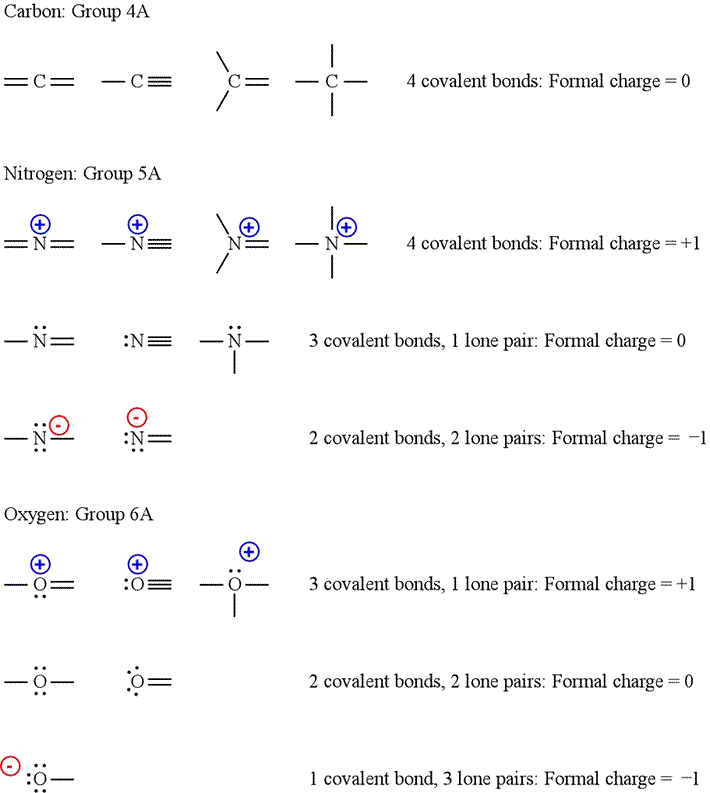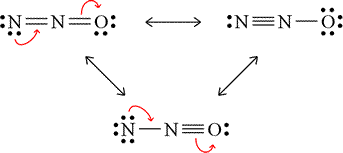CHEM 101
General Chemistry

J. D. Cronk    Syllabus    Topics

GENERAL CHEMISTRY TOPICS

### Formal charge

Rules for assigning formal charge to an atom in a Lewis structure. Patterns of formal charge for specific atom types. Using formal charge as a tool to assess quality of Lewis structures.

The rule or formula for assigning formal charge to atoms in Lewis structures is the following:

Formal charge = number of valence electrons - (number of lone-pair electrons + 1/2 number of bonding electrons)

Note that "lone pair electrons" are also known as "nonbonding pairs" or "unshared pairs".

Another rule that is very important to bear in mind is that the sum of formal charges of the Lewis structure of a molecule or ion must be equal to the net charge on the molecule or ion. If this is not the case, there is a mistake either in the formal charge assignments or in the Lewis structure (probably the wrong number of valence electrons).

#### Patterns of formal charge

Although we can easily calculate formal charge according to the formula above, it is helpful to be able to recognize patterns for selected elements. For example, carbon with four covalent bonds and no lone pairs has a formal charge of zero. Similarly, nitrogen with three covalent bonds and one lone pair and oxygen with two covalent bonds and two lone pairs both have formal charge of zero. Furthermore, for any element, converting a lone pair into a covalent bond changes the formal charge by plus one. Converting a covalent bond into a lone pair changes the formal charge by minus one.#### Using formal charge

The assignment of formal charge to the atoms in Lewis structures has a variety of uses. The relative contribution of non-equivalent resonance structures can be judged by a formal charge and electronegativity criterion. Negative formal charge should preferentially reside on more electronegative atoms, while positive formal charge is more readily borne by the relatively less electronegative atoms. Minimization of total formal charge is also in some contexts useful as a criterion for deciding the "best" Lewis structure.

Example: Assign formal charges to each of the non-equivalent resonance forms of dinitrogen monoxide, and predict their relative contribution to the resonance hybrid.The figure at left shows the non-equivalent resonance forms of dinitrogen monoxide. The arrows indicate movement of electron pairs that interconvert resonance forms (in clockwise sequence). Work out the formal charges on each atom in each of these forms. Try using both the formula and the patterns shown above to make the formal charge assignments. Then click on the figure to see the answer.

The formal charge assignments can be interpreted as follows. The top left form will contribute less to the resonance hybrid than the best form, on the top right. This is because the negative formal charge is carried by the less electronegative atom, nitrogen. Putting this formal charge on the oxygen, as in the form at top right, is preferred, since oxygen is more electronegative, and this form will contribute the most of the three to the actual resonance hybrid. The bottom form contributes the least, as it puts more negative charge on the less electronegative atom, a positive formal charge on the more electronegative atom, and the total formal charge is the greatest.